# Calculus 2 : Applications in Physics

## Example Questions

1 2 3 4 6 Next →

### Example Question #226 : Integrals

If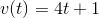and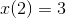, what is the original position function?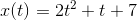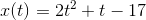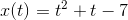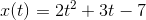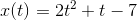Explanation:

Recall that the integral of the velocity function is the position function: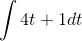Now, integrate: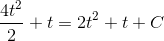Now plug in your initial conditions: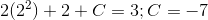### Example Question #227 : Integrals

If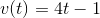and, what is the original position function?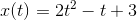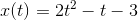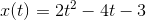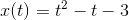Explanation:

Recall that the integral of velocity is the position function: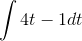Now, integrate. Remember to raise the exponent by 1 and also put that result on the denominator: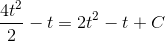Now plug in your initial conditions to solve for C: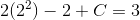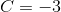### Example Question #228 : Integrals

If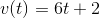and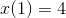, what is the original position function?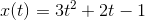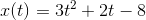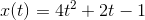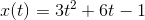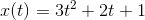Explanation:

Recall that the integral of the velocity function is the position function: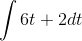Now, integrate. Remember to raise the exponent by 1 and also put that result on the denominator: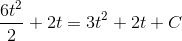Now, plug in your initial conditions: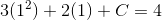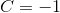### Example Question #229 : Integrals

In the vacuum of space, a particle is initially moving at a constant speed of only 6 meters/second away from you as you watch in your space capsule stationary relative to the particle. You track the object and then observe an acceleration that varies with time as,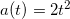After travelling for 20 minutes at this speed the particle is now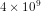km away from you. How far was it from you at the instant it began accelerating?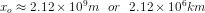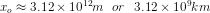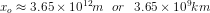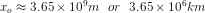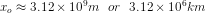Explanation:To solve the problem we first need to find the position function by successively integrating. First find the velocity function by integrating the acceleration: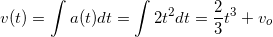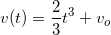The constant of integration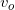is the initial velocity, which we are given as 6 meters-per-second. For convenience, we will omit the units in the equation.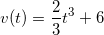Now we integrate the velocity function to find the position function: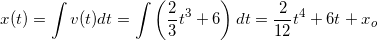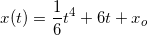The constant of integration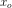represents the initial position when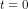. After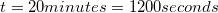the particle iskm, or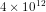meters, away. Using standard S.I. units (meters and seconds), solve forat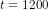seconds where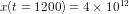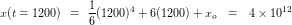Now solve for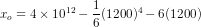1 2 3 4 6 Next →